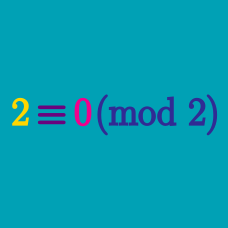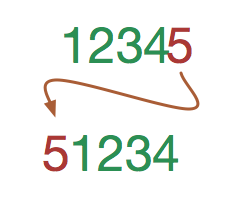Number Theory

# Modular Arithmetic Operations: Level 3 Challenges

What is the remainder when $1^{2013}+2^{2013}+\cdots +2012^{2013}+2013^{2013}$ is divided by $2014$?When we rotate an integer, we take the last digit (right most) and move it to the front of the number. For example, if we rotate $12345$, we will get $51234$.

What is the smallest (positive) integer $N$, such that when $N$ is rotated, we obtain $\frac{2}{3} N$?

Find the remainder when $6^{98}+8^{98}$ is divided by 98.

Find the smallest positive integer $k$ such that $1^2+2^2+3^2+\ldots+k^2$ is a multiple of 200.

${a^x \equiv a-2 \pmod{{\small(a-1)}}}$

If $a$ and $x$ are positive integers greater than 2, what is the value of $a?$

×

Problem Loading...

Note Loading...

Set Loading...